# SAT Math Multiple Choice Question 220: Answer and Explanation

### Test Information

Question: 220

10. What is the equation of a line that contains the point (6, -5) and has an x-intercept of 3 ?

• A.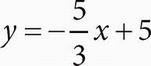• B.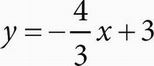• C.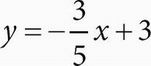• D.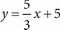A Plugging In the point (6, -5) in the answer choices could work, but it will likely make more than one equation true. The x-intercept is where the line crosses the x-axis, at the point where y = 0. Therefore, one point on the line is (3, 0). The choices are all in the slope-intercept form, y = mx + b, where m is the slope. To find the correct answer, calculate the slope of the line with the two points known to be on the line. The slope of a line is determined by the equation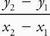. Therefore, the slope of the line shown can be calculated as follows: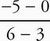=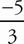. Only (A) has a slope of, so that is the correct answer.